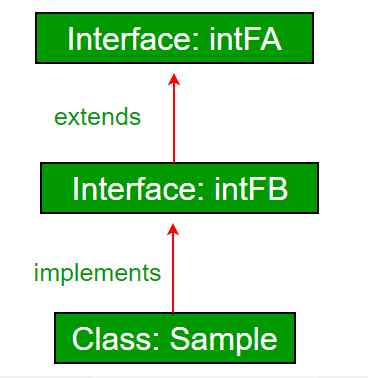GeeksforGeeks App
Open AppBrowser
Continue

## Related Articles

• Write an Interview Experience
• Java Tutorial
• Java Collection Tutorial

# Interfaces and Inheritance in Java

Prerequisites: Interfaces in Java, Java, and Multiple Inheritance A class can extend another class and/ can implement one and more than one interface.

Example:

## Java

 `// Java program to demonstrate that a class can``// implement multiple interfaces``import` `java.io.*;``interface` `intfA``{``    ``void` `m1();``}` `interface` `intfB``{``    ``void` `m2();``}` `// class implements both interfaces``// and provides implementation to the method.``class` `sample ``implements` `intfA, intfB``{``    ``@Override``    ``public` `void` `m1()``    ``{``        ``System.out.println("Welcome: inside the method m1");``    ``}` `    ``@Override``    ``public` `void` `m2()``    ``{``        ``System.out.println("Welcome: inside the method m2");``    ``}``}` `class` `GFG``{``    ``public` `static` `void` `main (String[] args)``    ``{``        ``sample ob1 = ``new` `sample();` `        ``// calling the method implemented``        ``// within the class.``        ``ob1.m1();``        ``ob1.m2();``    ``}``}`

Output;

```Welcome: inside the method m1
Welcome: inside the method m2```

Interface inheritance : An Interface can extend other interface.Inheritance is inheriting the properties of parent class into child class.
 Inheritance in Java is a mechanism in which one object acquires all the properties and behaviors of a parent object.
 The idea behind inheritance in Java is that you can create new classes that are built upon existing classes. When you inherit from an existing class, you can reuse methods and fields of the parent class.
 You can also add new methods and fields in your current class.
 Inheritance represents the IS_A relationship which is also known as parent- child relationship.

Eg:
Dog IS_A Animal
Car IS_A Vehicle
Employee IS_A Person
Surgeon IS_A Doctor etc.

## Java

 `class` `Animal``{``    ``public` `void` `eat()``    ``{``    ``}``}` `class` `Dog ``extends` `Animal``{``    ``public` `static` `void` `main(String args[])``    ``{``        ``Dog d=``new` `Dog();` `        ``d.eat();``    ``}``}`

Syntax of Java Inheritance

```class <Subclass-name> extends <Superclass-name>
{
//methods and fields
}```

Note: The extends keyword indicates that you are making a new class that derives
from an existing class. The meaning of “extends” is to increase the functionality.

Eg_1:

## Java

 `import` `java.io.*;``class` `Person {``    ``int` `id;``    ``String name;``    ``void` `set_Person()``    ``{` `        ``try` `{``            ``BufferedReader br = ``new` `BufferedReader(``                ``new` `InputStreamReader(System.in));``            ``System.out.println(``"Enter the Id:"``);``            ``id = Integer.parseInt(br.readLine());``            ``System.out.println(``"Enter the Name"``);``            ``name = br.readLine();``        ``}``        ``catch` `(Exception ex) {``            ``ex.printStackTrace();``        ``}``    ``}``    ``void` `disp_Person()``    ``{``        ``System.out.print(id + ``"\t"` `+ name + ``"\t"``);``    ``}``}``class` `Employee ``extends` `Person {``    ``int` `sal;``    ``String desgn;``    ``void` `set_Emp()``    ``{``        ``try` `{``            ``set_Person();``            ``BufferedReader br = ``new` `BufferedReader(``                ``new` `InputStreamReader(System.in));``            ``System.out.println(``"Enter the Designation:"``);``            ``desgn = br.readLine();``            ``System.out.println(``"Enter the Salary:"``);``            ``sal = Integer.parseInt(br.readLine());``        ``}``        ``catch` `(Exception ex) {``            ``ex.printStackTrace();``        ``}``    ``}``    ``void` `disp_Emp()``    ``{``        ``disp_Person();``        ``System.out.println(desgn + ``"\t"` `+ sal);``    ``}``    ``public` `static` `void` `main(String args[])``    ``{``        ``Employee e1 = ``new` `Employee();``        ``e1.set_Emp();``        ``e1.disp_Emp();``    ``}``}`

Eg_2:

## Java

 `class` `Person1 {``    ``int` `id;``    ``String name;``    ``void` `set_Person(``int` `id, String name)``    ``{``        ``try` `{``            ``this``.id = id;``            ``this``.name = name;``        ``}``        ``catch` `(Exception ex) {``            ``ex.printStackTrace();``        ``}``    ``}``    ``void` `disp_Person()``    ``{``        ``System.out.print(id + ``"\t"` `+ name + ``"\t"``);``    ``}``}``class` `Employee1 ``extends` `Person1 {``    ``int` `sal;``    ``String desgn;``    ``void` `set_Emp(``int` `id, String name, String desgn, ``int` `sal)``    ``{``        ``try` `{``            ``set_Person(id, name);``            ``this``.desgn = desgn;``            ``this``.sal = sal;``        ``}``        ``catch` `(Exception ex) {``            ``ex.printStackTrace();``        ``}``    ``}``    ``void` `disp_Emp()``    ``{``        ``disp_Person();``        ``System.out.print(desgn + ``"\t"` `+ sal);``    ``}``    ``public` `static` `void` `main(String args[])``    ``{` `        ``Employee1 e1 = ``new` `Employee1();``        ``e1.set_Emp(``1001``, ``"Manjeet"``, ``"AP"``, ``20000``);``        ``e1.disp_Emp();``    ``}``}`

Types of inheritance in java

 Java Support three types of inheritance in java: single level, multilevel and
hierarchical inheritance in case of classes to avoid ambiguity.
 In java programming, multiple and hybrid inheritance is supported through
interface only.

Single Inheritance Example

When a class inherits another class, it is known as a single inheritance.

## Java

 `class` `A {``    ``int` `a;``    ``void` `set_A(``int` `x) {``      ``a = x;``    ``}``}``class` `B ``extends` `A {``    ``int` `b, product;``    ``void` `set_B(``int` `x) {``      ``b = x;``    ``}``    ``void` `cal_Product()``    ``{``        ``product = a * b;``        ``System.out.println(``"Product ="` `+ product);``    ``}``    ``public` `static` `void` `main(String[] args)``    ``{``        ``B b = ``new` `B();``        ``b.set_A(``5``);``        ``b.set_B(``5``);``        ``b.cal_Product();``    ``}``}`

Multilevel Inheritance Example

When there is a chain of inheritance, it is known as multilevel inheritance.

## Java

 `class` `A {``    ``int` `a;``    ``void` `set_A(``int` `x) {``      ``a = x;``    ``}``}``class` `B ``extends` `A {``    ``int` `b;``    ``void` `set_B(``int` `x) {``      ``b = x;``    ``}``}``class` `C ``extends` `B {``    ``int` `c, product;``    ``void` `cal_Product()``    ``{``        ``product = a * b;``        ``System.out.println(``"Product ="` `+ product);``    ``}``    ``public` `static` `void` `main(String[] args)``    ``{``        ``C c = ``new` `C();``        ``c.set_A(``5``);``        ``c.set_B(``5``);``        ``c.cal_Product();``    ``}``}`

Hierarchical Inheritance Example
When two or more classes inherit a single class, it is known as hierarchical
inheritance.
Eg:

## Java

 `class` `A {``    ``int` `a;``    ``void` `set_A(``int` `x) {``      ``a = x;``    ``}``}``class` `B ``extends` `A {``    ``int` `b;``    ``void` `set_B(``int` `x) {``      ``b = x;``    ``}``}``class` `C ``extends` `A {``    ``int` `c;``    ``void` `set_C(``int` `x) {``      ``c = x;``    ``}`

## Java

 `// Java program to demonstrate inheritance in``// interfaces.``import` `java.io.*;``interface` `intfA {``    ``void` `geekName();``}` `interface` `intfB ``extends` `intfA {``    ``void` `geekInstitute();``}` `// class implements both interfaces and provides``// implementation to the method.``class` `sample ``implements` `intfB {``    ``@Override` `public` `void` `geekName()``    ``{``        ``System.out.println(``"Rohit"``);``    ``}` `    ``@Override` `public` `void` `geekInstitute()``    ``{``        ``System.out.println(``"JIIT"``);``    ``}` `    ``public` `static` `void` `main(String[] args)``    ``{``        ``sample ob1 = ``new` `sample();` `        ``// calling the method implemented``        ``// within the class.``        ``ob1.geekName();``        ``ob1.geekInstitute();``    ``}``}`

Output:

```Rohit
JIIT```

An interface can also extend multiple interfaces.

## Java

 `// Java program to demonstrate multiple inheritance``// in interfaces` `import` `java.io.*;` `interface` `intfA {``    ``void` `geekName();``}` `interface` `intfB {``    ``void` `geekInstitute();``}` `interface` `intfC ``extends` `intfA, intfB {``    ``void` `geekBranch();``}` `// class implements both interfaces and provides``// implementation to the method.``class` `sample ``implements` `intfC {``    ``public` `void` `geekName() { System.out.println(``"Rohit"``); }` `    ``public` `void` `geekInstitute()``    ``{``        ``System.out.println(``"JIIT"``);``    ``}` `    ``public` `void` `geekBranch() { System.out.println(``"CSE"``); }` `    ``public` `static` `void` `main(String[] args)``    ``{``        ``sample ob1 = ``new` `sample();` `        ``// calling the method implemented``        ``// within the class.``        ``ob1.geekName();``        ``ob1.geekInstitute();``        ``ob1.geekBranch();``    ``}``}`

Output

```Rohit
JIIT
CSE```

Why Multiple Inheritance is not supported through a class in Java, but it can be possible through the interface? Multiple Inheritance is not supported by class because of ambiguity. In the case of interface, there is no ambiguity because the implementation of the method(s) is provided by the implementing class up to Java 7. From Java 8, interfaces also have implementations of methods. So if a class implements two or more interfaces having the same method signature with implementation, it is mandated to implement the method in class also. Refer to Java and Multiple Inheritance for details.

This article is contributed by Nitsdheerendra. If you like GeeksforGeeks and would like to contribute, you can also write an article using write.geeksforgeeks.org or mail your article to review-team@geeksforgeeks.org. See your article appearing on the GeeksforGeeks main page and help other Geeks.